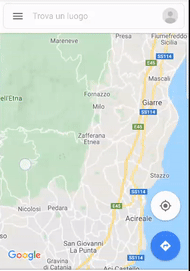# Android QuestionSearch for points of interest within a map section

#### Star-Dust

##### Expert
I need to make a particular application. And I need to know if it can be done with the Google Map API and possibly how.

I have a list of addresses (or points of interest) with EXCEL that I imported on google maps. (I also made KML / KMZ files that I use on Google Earth)

Of these addresses I need to know which ones are in a map section, similar to what is shown in the image below.Obviously I don't have to draw free the borders by hand but I have a KML file containing the boundaries to which the area of my interest is enclosed.

I would like to know which of the addresses are within the area of interest, which is generally a trapezoid.

Is it possible to do it and how?

Longtime User

#### Star-Dust

##### Expert
Thanks, I'll try to understand something

#### lucasheer

##### Active Member
Longtime User
If you're looking for a radius/distance calculation:

B4X:
``````Private Sub deg2rad(deg As Double) As Double
Return (deg * cPI / 180.0)
End Sub
Return rad / cPI * 180.0

End Sub

Public Sub distance(lat1 As Double, lon1 As Double, lat2 As Double, lon2 As Double, unit As Char) As Double
If lat1 = lat2 And lon1 = lon2 Then
Return 0
Else
Dim theta As Double = lon1 - lon2
dist = ACos(dist)
dist = dist * 60 * 1.1515
If unit = "K" Then
dist = dist * 1.609344
Else If unit = "N" Then
dist = dist * 0.8684
End If
Return dist
End If
End Sub``````

I did a couple of calculations:
B4X:
``````    Log( distance(35.8209082, -90.8088206, 35.1288636, -90.2509744, "M")) 'Jonesboro AR to Memphis TN : 57 miles
Log( distance(35.8209082, -90.8088206, 37.7048359, 14.9922625, "M")) 'Jonesboro AR to Zafferana : 5488 miles``````

You could add only the ones that are <80 miles. I will not be going to Zafferana since it is not <80 miles..#### Star-Dust

##### Expert
I'm not looking for a search within a radius ma.allibyerbo I do a polygon

#### lucasheer

##### Active Member
Longtime User
I'm not looking for a search within a radius ma.allibyerbo I do a polygon

The circle drawing threw me off lol

#### lucasheer

##### Active Member
Longtime User
I'm not looking for a search within a radius ma.allibyerbo I do a polygon

Here is a bit of a different approach. Works with triangles, squares, pentagons, hexagons, etc.. It will only work with convex shapes.

B4X:
``Type Point (X As Double, Y As Double)``
B4X:
``````Public Sub IsPointInPolygon(p As Point, pList() As Point) As Boolean
Dim minX As Double = pList(0).X
Dim maxX As Double = pList(0).X
Dim minY As Double = pList(0).Y
Dim maxY As Double = pList(0).Y

For b=1 To pList.length - 1
Dim q As Point = pList(b)
minX = Min(q.X, minX)
maxX = Max(q.X, maxX)
minY = Min(q.Y, minY)
maxY = Max(q.Y, maxY)
Next

If p.X < minX Or p.X > maxX Or p.Y < minY Or p.Y > maxY Then
Return False
End If

Dim inside As Boolean = False
Dim i As Int = 0
Dim j As Int = pList.length - 1

Do While i < pList.length - 1

Dim iPoint As Point = pList(i)
Dim jPoint As Point = pList(j)

If ((iPoint.Y > p.Y) <> (jPoint.Y > p.Y) And p.X < (jPoint.X - iPoint.X) * (p.Y - iPoint.Y) / (jPoint.Y - iPoint.Y) + iPoint.X) Then
inside = (inside = False)
End If

i = i + 1
j = Min(i, i - 1)

Loop
Return inside
End Sub``````

B4X:
``Log("IS INSIDE: " & IsPointInPolygon(getPoint(25,1), Array As Point(getPoint(25, 0),getPoint(50,50),getPoint(0,50))))``

Not sure if this would work with lat/lon points lol

#### Star-Dust

##### Expert
It is an interesting algorithm for locating a point within a polygon.

I was wondering if something already did this in the GOGLE API. So you ultimately calculate it.

#### lucasheer

##### Active Member
Longtime User
It is an interesting algorithm for locating a point within a polygon.

I was wondering if something already did this in the GOGLE API. So you ultimately calculate it.

I found that function on Google and just translated/tested itI had to work on a couple of property/reality apps, and they stored the Lat,Lon of the properties, and showed them only if the distance is within the search threshold.

I'm not sure if there's anything in the API to do this though. I might research it

#### DonManfred

##### Expert
Longtime User
You can use the PolyUtil.containsLocation method from the Google Maps Android API Utility Library. From the documentation:
public static boolean containsLocation(LatLng point, java.util.List polygon, boolean geodesic)

Computes whether the given point lies inside the specified polygon. The polygon is always considered closed, regardless of whether the last point equals the first or not. Inside is defined as not containing the South Pole -- the South Pole is always outside. The polygon is formed of great circle segments if geodesic is true, and of rhumb (loxodromic) segments otherwise.

Source PolyUtil.

I guess this should fit your requirements perfectly.

Last edited:
•Star-Dust

#### Star-Dust

##### Expert
I found that function on Google and just translated/tested itI had to work on a couple of property/reality apps, and they stored the Lat,Lon of the properties, and showed them only if the distance is within the search threshold.

I'm not sure if there's anything in the API to do this though. I might research it
You did a great tradution, but I remembered that there was an api or something already studied by Google.

So I avoid turning the POI addresses into coordinates

Replies
4
Views
935
Replies
5
Views
482
Replies
1
Views
307
Replies
1
Views
2K
Android Question Android Printing Framework
Replies
27
Views
11K